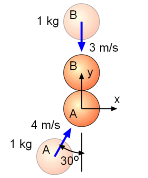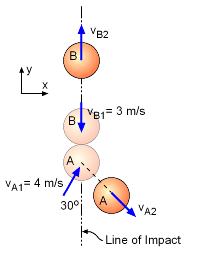Ch 4. Particle Momentum and Impulse Multimedia Engineering Dynamics Impulse & Momentum Consv. Linear Momentum Impact AngularMomentum MassFlow
 Chapter - Particle - 1. General Motion 2. Force & Accel. 3. Energy 4. Momentum - Rigid Body - 5. General Motion 6. Force & Accel. 7. Energy 8. Momentum 9. 3-D Motion 10. Vibrations Appendix Basic Math Units Basic Equations Sections Search eBooks Dynamics Fluids Math Mechanics Statics Thermodynamics Author(s): Kurt Gramoll ©Kurt GramollDYNAMICS - EXAMPLECollision of Two Balls Example Two balls, A and B, impact each other as shown. If the coefficient of restitution, e, is 1.0 (perfectly elastic), what is the velocity magnitude of ball B after impact? SolutionVelocity of Balls Before and After Collision Ball B only has a velocity in the y-direction, so first consider y-direction momentum. Conservation of momentum before and after impact gives      mAvA1y + mBvB1y = mAvA2y + mBvB2y      4 cos30 - 3 = -vA2y + vB2y      0.4641 = -vA2y + vB2y There are still unknowns, so the impact equation needs to be used,      e = (vB2y - vA2y)/(vA1y - vB1y)      1 = [vB2y - (-vA2y)] / [4 cos30 - (-3)]      3.464 + 3 = vB2y + vA2y      6.464 = vB2y + vA2y Combining both equations      0.4641 = -(6.464 - vB2y) + vB2y      0.4641 + 6.464 = 2vB2y      vB2y = 3.464 m/s vB2y = vB2 = 3.464 m/s

Practice Homework and Test problems now available in the 'Eng Dynamics' mobile app
Includes over 400 problems with complete detailed solutions.
Available now at the Google Play Store and Apple App Store.